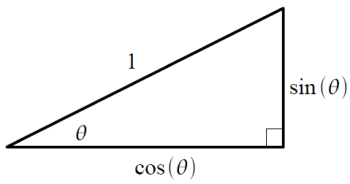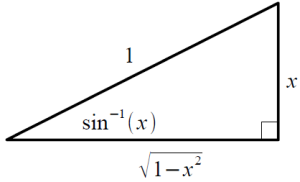## Inverse Trig Derivatives

### Method 1

This is a nice trick for calculating the derivatives of inverse trig functions.  I’ll demonstrate the process for the  derivative of $$\sin^{-1}\theta$$.  The rest of the inverse trig derivatives can be found using the same method.

First, recall the definitions of the trig functions on the unit circle (Figure 1 below)Figure 1

The inverse sine function is defined by the property $$!\displaystyle \sin^{-1}(\sin\theta)=\theta$$ If we define $$!\displaystyle x=\sin \theta\tag{1}$$ we can re-label the diagram in terms of $$x$$ (Figure 2)Figure 2

The important thing to note is that $$!\displaystyle \theta=\sin^{-1} (x).$$ Differentiating Eq. (1) with respect to $$x$$ yields $$!\displaystyle 1= \cos \theta \frac{d\theta}{dx}$$ The derivative is then, $$!\displaystyle \frac{d\theta}{dx}= \frac{1}{\cos \theta}.$$ From Figure 2, we see $$!\displaystyle \cos \theta=\sqrt{1-x^2}.$$ Using this, we can write the derivative in terms of $$x$$, $$!\displaystyle \frac{d\theta}{dx}= \frac{d}{dx}\sin^{-1}(x)= \frac{1}{\sqrt{1-x^2}},$$ and thus we have found the derivative of the inverse sine!  The same method can be used to find all of the other inverse trig derivatives.  You have to think about what should be labeled $$x$$, but it is rather straightforward.  It is then possible to construct a table of inverse trig derivatives, which can be used as an integral table. For instance $$!\displaystyle \int \frac{dx}{\sqrt{1-x^2}}=\sin^{-1}(x) + C$$ which can be generalized to $$!\displaystyle \int \frac{dx}{\sqrt{a^2-x^2}}=\sin^{-1}(x/a) + C$$ where $$a$$ is a constant.

### Method 2

It is also possible to compute the derivative of an inverse trig function directly, using an explicit expression for the inverse function. The explicit expressions are listed in reference books and various web resources. You can also derive the expressions yourself. Refer to my page on Inverse Trig Functions for more information.

For example, the inverse sine is given by $$!\displaystyle\sin^{-1}(x)=-i\ln\left(ix+\sqrt{1-x^2}\right).$$ So, the derivative is $$!\displaystyle\frac{d}{dx}\sin^{-1}(x)=\frac{d}{dx}\left[-i\ln\left(ix+\sqrt{1-x^2}\right)\right].$$ After performing the differentiation and simplifying, the result is $$!\displaystyle\frac{d}{dx}\sin^{-1}(x)=\frac{1}{\sqrt{1-x^2}}.$$